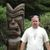# Get new point coordinate by current Point coordinate, angle and distance

819
6
07-20-2021 07:57 AMNew Contributor

Hello all,

i want to create new point to be away by specific angle and distance from existing point .

i checked geometryEngine.offset but its working only for polyline or polygon not working for points also i need to control the angle so i create the new point up or down not only right and left.

Thank you all

1 Solution

Accepted SolutionsbyEsri Regular Contributor

I have some code that uses latitude and longitude as input values to calculate a second point based on bearing and distance. Haven't used this in quite a while. I'm not sure how much efficiency you need, you would have handle any additional coordinate conversions:

``````        function bearingDistance(lat, lon, radius, bearing){
const R_KM = 6371; // radius in KM
const d = radius/R_KM; //angular distance on earth's surface

);

);

return {
}
}

return degrees * Math.PI / 180;
}

return radians * 180 / Math.PI;
}``````

6 RepliesbyEsri Regular Contributor

I have some code that uses latitude and longitude as input values to calculate a second point based on bearing and distance. Haven't used this in quite a while. I'm not sure how much efficiency you need, you would have handle any additional coordinate conversions:

``````        function bearingDistance(lat, lon, radius, bearing){
const R_KM = 6371; // radius in KM
const d = radius/R_KM; //angular distance on earth's surface

);

);

return {
}
}

return degrees * Math.PI / 180;
}

return radians * 180 / Math.PI;
}``````New Contributor III

Hi sir, we are using the same code as given but getting the point at a wrong distance, kindly help us how to get the new point at given distance.

For lat- lattittue

log- logitude

radius- distance we are passing(line distance)

bearing- angle

if we enter 200 mt distance, the line length will be 652 mt.  How to get the new point at the exact line distance, which we are providing.  The screen shot provided for reference.byEsri Regular ContributorNew Contributor III

thank you sir,New Contributor

its working, thank you exactly what i want :grinning_face:byEsri Regular Contributor

You can also try the pointFromDistance() method: Geodetically computes the location at a defined distance and direction from a known location.